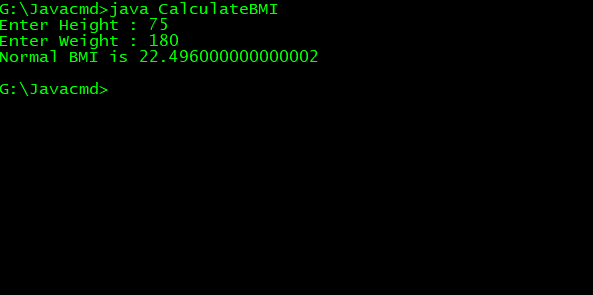## Calculate BMI Javacalculate bmi java

Write a Java application with the following prototypes that returns the user's body mass index (BMI)

public static double calculate BMI(double weight, double height)

To calculate BMI based on weight in pounds (lb) and height in inches (in), use this formula:

and

public static String findStatus(double bmi)

Categorizes it as underweight, normal, overweight, or obese, based on the table from the United States Centers for Disease Control:

 BMI Weight Status Below 18.5 Underweight 18.5 – 24.9 Normal 25.0-29.9 Overweight 30.0 and above Obese

Prompt the user to enter weight in pounds and height in inches.

`import java.util.Scanner;class CalculateBMI{    public static double calculateBMI(double height, double weight)    {        double bmi = 703*(weight/(height*height));        if (bmi < 18.5)        {            System.out.println("Under Weight BMI is " + bmi);        }        else if (bmi >=18.5 && bmi <= 24.9 )        {            System.out.println("Normal BMI is " + bmi);        }        else if (bmi >=25.0 && bmi <= 29.9 )        {            System.out.println("Over Weight BMI is " + bmi);        }        else if (bmi >=30.0 )        {            System.out.println("Obese BMI is " + bmi);        }        return bmi;    }    public static void main(String arg[]){        Scanner user_input =new Scanner(System.in);        System.out.print("Enter Height : ");        double height = user_input.nextDouble();        System.out.print("Enter Weight : ");        double weight = user_input.nextDouble();        calculateBMI(height, weight);    }}`